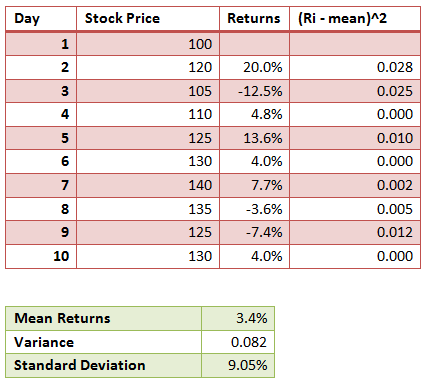# Variance and Standard Deviation

Risk is the possibility that actual returns might differ, or vary, from expected returns. In fact, actual returns will most likely differ from expected returns. It is important for decision-makers to estimate the magnitude and likelihood of the difference between actual and estimated returns. After all, there is a big difference if your predictions result in an error of only $100 versus an error of$1 million.

By using the concepts of variance and standard deviation, investors can judge not only how wrong their estimates might be, but also estimate the likelihood, or probability, of favorable or unfavorable outcomes. With the tools of expected return and standard deviation, financial decision-makers are better able to evaluate alternative investments based on risk-return tradeoffs, and their own risk preferences.

In general, the risk of an asset or a portfolio is measured in the form of the standard deviation of the returns, where standard deviation is the square root of variance. Let’s look at how standard deviation and variance is calculated.

Let’s say our observation data set is the returns data of a stock. Using this data, we calculate the mean/average returns. The variance of the asset returns will then be the average of the square of the difference between the returns and the mean.The standard deviation will simply be the square root of the variance.

The following is a simple example that illustrates the calculation:Note that in the above example we start with the stock price. Once we have the stock price data, the first step is to calculate the returns. What kind of returns we have depends on the periodicity of the data. For example, if we have daily prices, then we calculate the daily returns, which is calculated as (P(t1) – P(t0) /P(t0).

Notations

The population variance and standard deviation are written as s2 and s while the sample variance and standard deviation are written as s2 and s. For sample variance, we use n-1 in the denominator instead of N. This is done because as per the theory, using the entire sample set n, will underestimate the population variance.